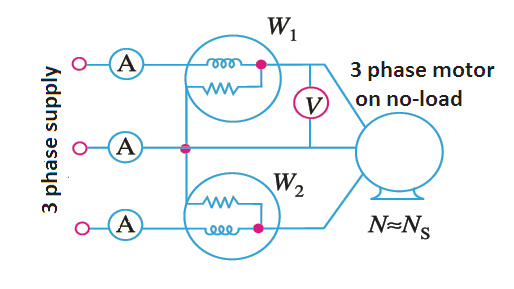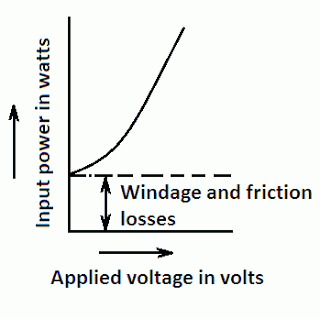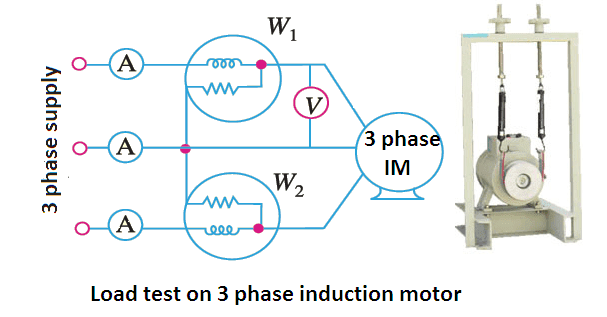# No Load Test of Induction Motor

The no load test and the blocked rotor test are two main induction motor tests, which are performed on induction motor to know the different losses, power factor and efficiency of the induction motor.

# No Load Test of Induction MotorThe no load test of 3 phase induction motor is performed on induction motor when it is running without load. This test tells us the magnitude of constant losses occurring in the motor.

The set up for no load test of induction motor is shown in the figure. The machine is started in the usual way and runs unloaded from normal voltage mains. On the mains side suitable instruments are connected between supply mains and motor terminals to measure power, line current and line voltage.

For power and power factor measurement, two single phase watt meters are used. Since the motor is running without load, p.f. of the motor is low less than 0.5, so one of the watt meters will give negative reading. Total power drawn by the motor is the difference of the two wattmeter readings.

To understand the concept properly, you should read also the power measurement in three phase circuits by two watt meter method.

The signs of readings are taken with various values of applied voltage and then curve is plotted against power and input voltage. From this curve windage and friction losses are determined.

Since the motor is not loaded so input power absorbed by the motor is providing losses only. Losses are occurring in iron core of the stator as well as the rotor which are called core losses. A small amount of copper loss is also occurring in stator winding. This can be neglected since the stator current is very small.

Therefore, total power consumed = rotor iron loss + stator iron loss + copper loss in stator + friction and windage loss

The readings noted at normal voltage, and at rated frequency are considered to find out the fixed core losses.

## Calculation from No-Load Test of Induction Motor

The procedure to find out the separate losses from no-load test of an induction motor is as follows:

Total power by two watt meter = Po watts.

Copper losses in the stator = 3Io2R1.

Where Io is the no-load current measured by an ammeter at normal voltage and rated frequency of supply, R1 is the stator winding resistance and V per phase is the applied voltage.

Therefore, total constant losses = (Po – 3Io2R1) watts.Now to determine the friction and windage losses the curve drawn between applied voltage and input power is extended until it cuts, the vertical axis.

The point where it intersects, is the zero applied voltage. When applied voltage is zero the core losses and stator copper losses are zero.

Therefore, power input at no load and zero voltage applied represents the windage and friction losses.

Other calculations are as follows:

No-load power factor cos φo = Po /3IoV

No-load reactance Xo = V/Iosin φo

# Blocked Rotor Test on Induction MotorThe set up for the blocked rotor test on induction motor is shown in the figure. It is performed by locking the rotor (by keeping the rotor not to rotate).

This is carried out to know the copper losses, power factor at short circuit current; total equivalent resistance and reactance.

This test is just similar to short circuit test of the transformer.

Starting with zero voltages across the stator, the applied voltage is gradually increased in steps until the full load current is circulated. The readings of voltmeter ammeter and watt meters are noted. While performing this test the following points are taken care of:

• Means of holding tight (not to rotate) the rotor should be of proper strength.
• The direction of rotation of a rotor should be established prior to start the test and direction of force, which is to keep the rotor blocked (unmoved) should be in opposite direction.
• As the windings get heated the test should be carried out quickly.
• The short circuit current should not be more than the full load current.

## Calculations from Blocked Rotor Test of Induction Motor

Let Psc is the total power measured when Isc/phase is the current circulating and Vsc/phase is the voltage applied.

Then equivalent impedance/phase, Z1’ = Vsc/Isc  ohm

Power factor, cos φsc = Psc/3VscIsc

Let R1’ be the equivalent resistance then
R1’ = Psc/3I2sc ohm

Therefore, equivalent reactance,
X1’ = √ [(Z1’)2– (R1’)2].

The whole power input to the motor when the rotor is locked is absorbed as full copper losses in the motor as well as minute iron losses. A small voltage only (10 to 15% of normal voltage) is applied to circulate full load current in the motor.

Since iron losses depend on supply voltage and very small supply voltage is applied in load test of induction motor are therefore these losses are very small and hence these are neglected.

Therefore, Psc = total copper losses in motor.

If Isc = full load current of motor, then Psc is total copper loss on full load.

# Load Test of Induction Motor

To determine how speed, efficiency, power factor, stator current, torque and slip of an induction motor vary with load, the load test on an induction motor is performed.

The motor is loaded with break pully arrangement and the effect of increasing load on the above quantities observed and a graph is plotted between the load (on X-axis) above quantities (on Y-axis).